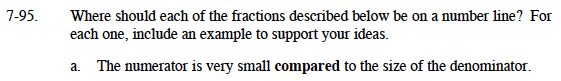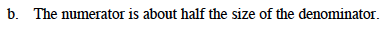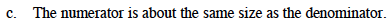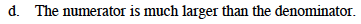### Home > MC1 > Chapter 7 > Lesson 7.2.5 > Problem7-95

7-95.A fraction becomes smaller as the denominator gets bigger and the numerator gets smaller.Consider the fraction five-eighths, which is very close to four-eighths. What is another way to write four-eighths?

If the number is about half the size of the denominator the fraction should be close to

$\frac{1}{2} \text{ on the number line.}$Think about the Giant Ones. How does the numerator relate to the denominator?
How far would the number move on the number line if the numerator were one integer bigger or smaller?This situation is opposite from part (a). Consider your answer to part (a) as you answer this problem.

Its position will be much larger than 1.